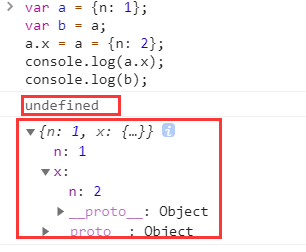﻿ 几个例子理解不同数据类型的堆栈内存处理-资讯-Jquery在线

## js代码的运行环境

• 浏览器 内核（引擎）
• node
• webview（hybrid，嵌入到手机app里面，在app里面运行）
• ...

## js如何运行（示例1）

``````var a = 12;
var b = a;
b = 13;
console.log(a);
``````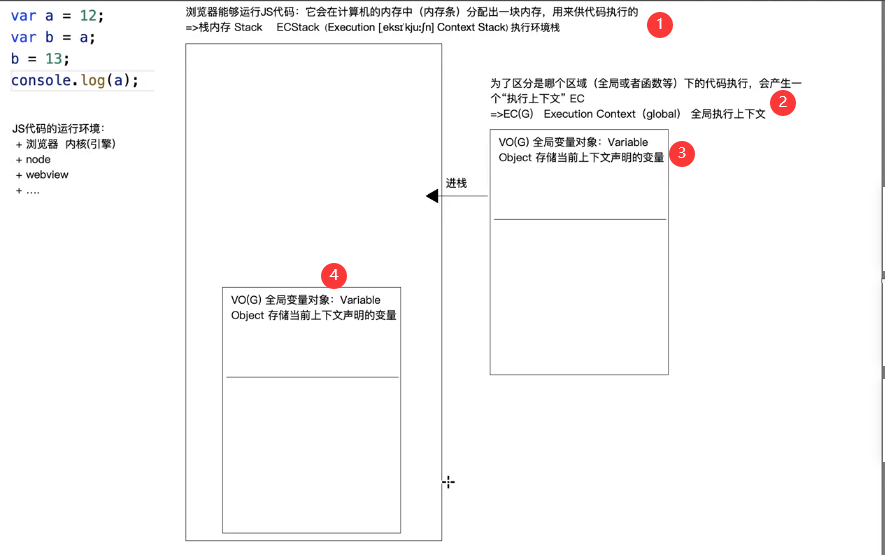### 声明变量的步骤

var [变量] = [值]

1. 先创建值（执行等号右边）
• 基本数据类型是直接存储在栈内存当中
• 引用类型的值，都是开辟一个单独的内存空间（堆内存Heap）存储信息
2. 声明变量 declare
• 存放到当前上下文的变量对象中（VO/AO）
3. 定义（赋值）变量：让变量和值关联到一起，也就是所谓的赋值操作，也叫定义（defined）或指针指向
• 所以`var n; //默认值是undefined 未定义`

1. 在内存中开辟空间，存储`12`
2. 声明变量`a`
3. `12`赋值给`a`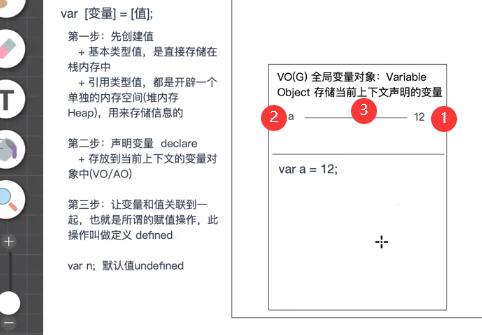`var b = a`处理是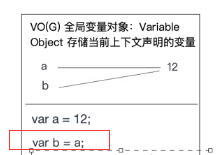`b=13`的处理步骤是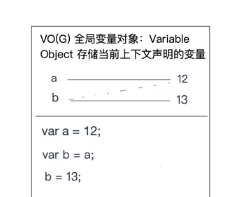1. 在内存中开辟空间，存储`13`
2. 因为`b`已经在当前上下文的变量对象中，所以不需要第二步声明
3. `13`赋值给`b`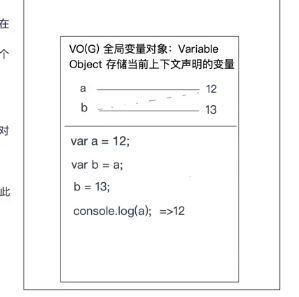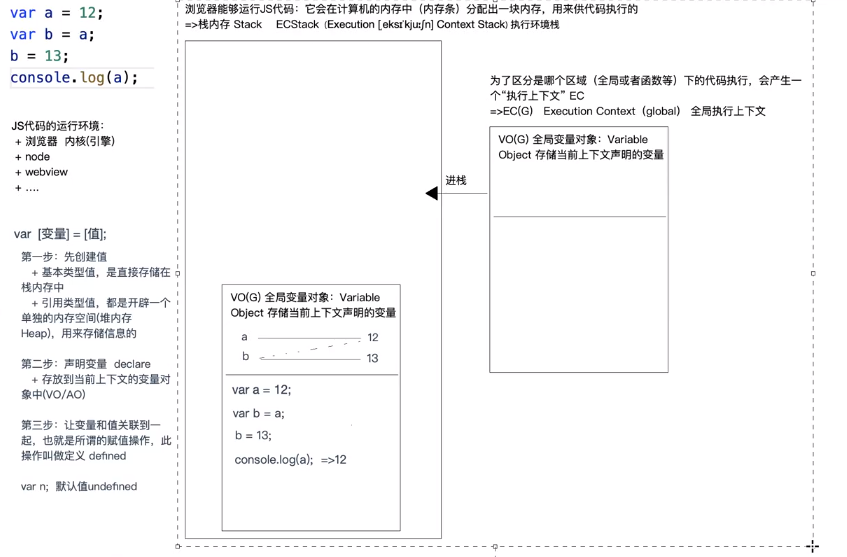## js如何运行（示例2）

``````var a = {n: 12};
var b = a;
b['n'] = 13;
console.log(a.n);
``````

• 在计算机内存中分配一个单独的内存出来（堆内存 Heap）
• 这块堆内存有一个16进制的地址用来寻找
• 把对象中的键值对分别存储到堆内存当中
• 把堆内存地址放置到栈中，供变量调用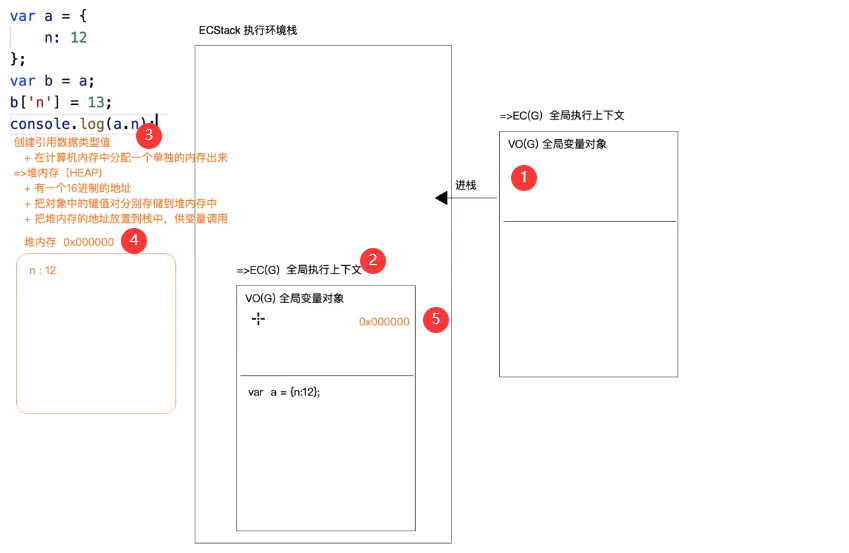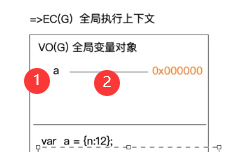`var b = a;`时，因为`a`为 变量，所以不需要创建值，接着声明`b`，最后赋值，将栈中`a`指向的地址也同样赋值给`b`，让`b`也指向那个16进制地址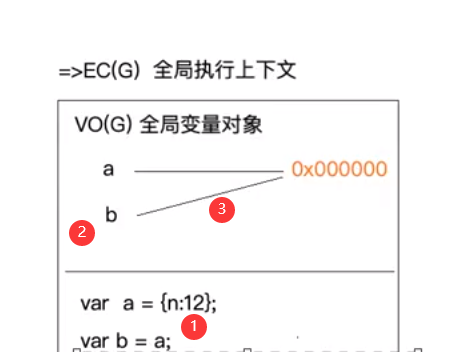`b['n'] = 13`运行原理：

`b['n'] = 13`属于对象的成员访问

• `b`首先基于地址`0x000000`找到堆内存
• 把堆内存中成员为`n`的值改为`13`
• `console.log(a.n)`也属于成员访问 所以输出`13`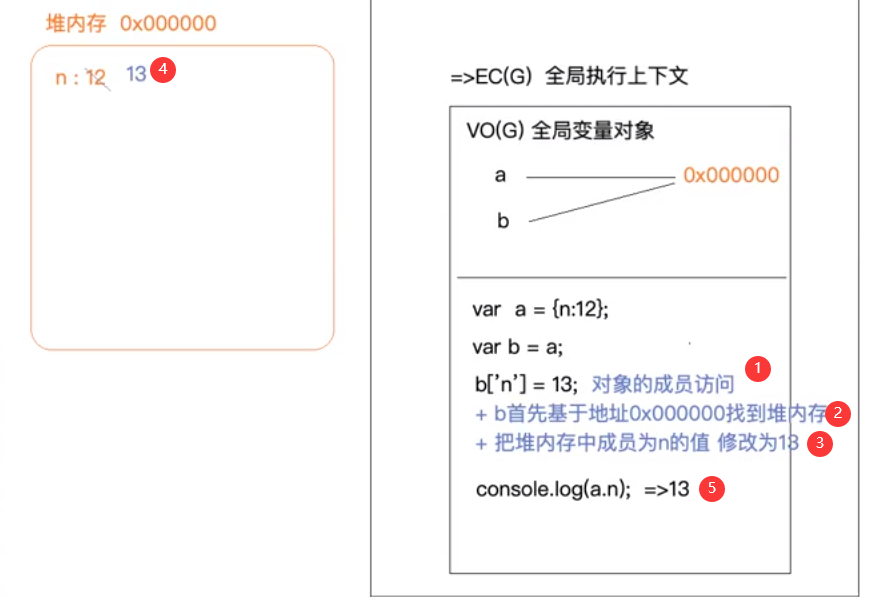## js如何运行（示例3）

``````var a = {n: 12};
var b = a;
b = {n: 13};
console.log(a.n);
``````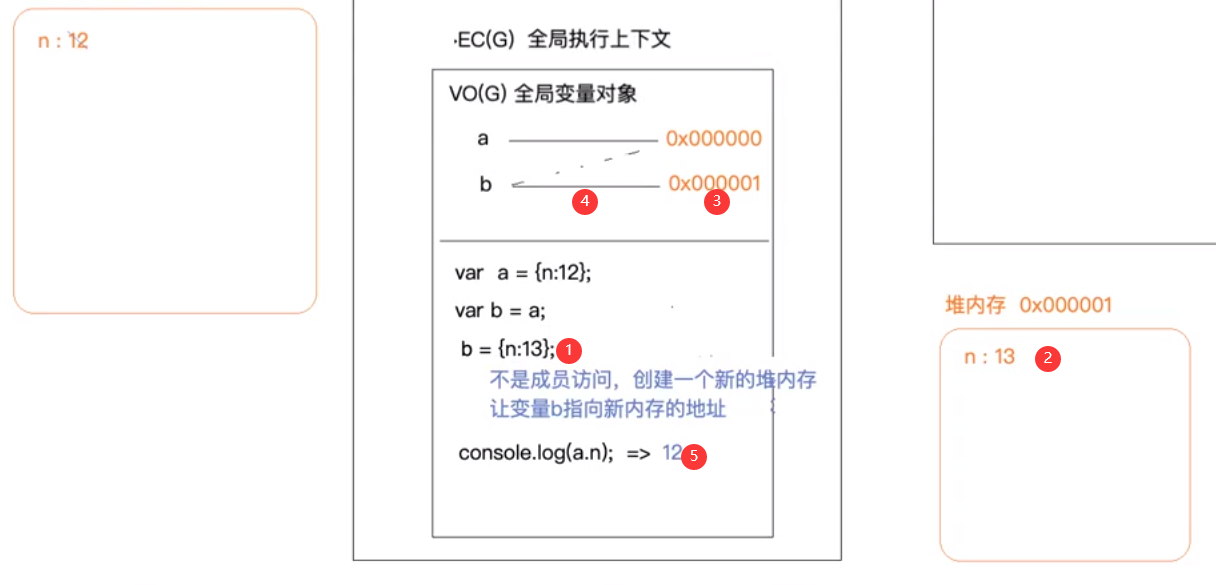### GO global object 全局对象

GO global object 全局对象，是加载页面默认形成的。在浏览器中，加载页面时，在全局上下文中会默认定义一个叫`window`的对象，其中有`setTimeout`,`setInterval`等供js调用的属性和方法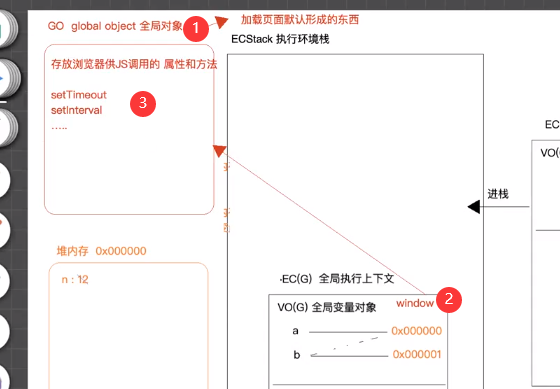JavaScript 中有一个特殊的对象，称为全局对象（Global Object），它及其所有属性都可以在程序的任何地方访问，即全局变量

`global``process``__filename``__dirname`

## js如何运行（示例4）

``````var a = {n: 1};
var b = a;
a.x = a = {n: 2};
console.log(a.x);
console.log(b);
``````
``````var a = {n: 1};
var b = a;
``````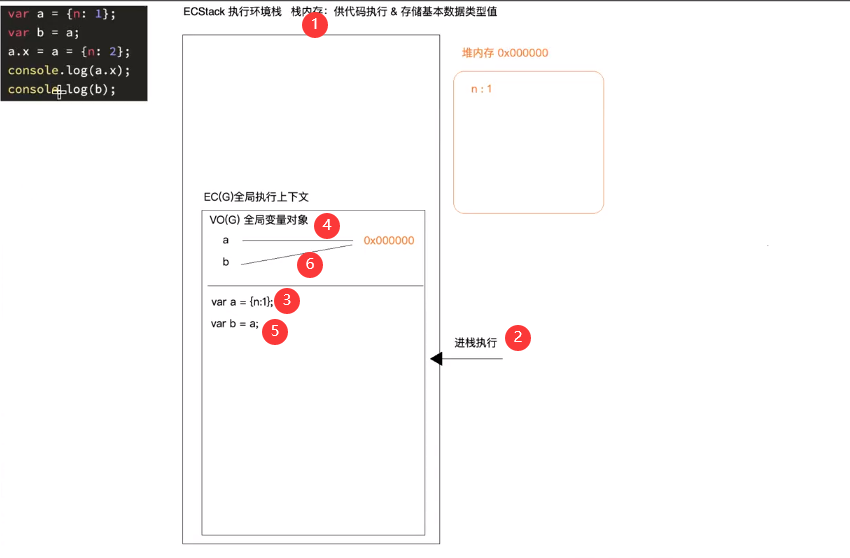``````var a=12,b=13;
``````

``````var a=12
var b=13
``````
``````var a=b=13
``````

``````b=13
var a=b//或者var a=13
``````

`a.x = a = {n: 2};`运算步骤如下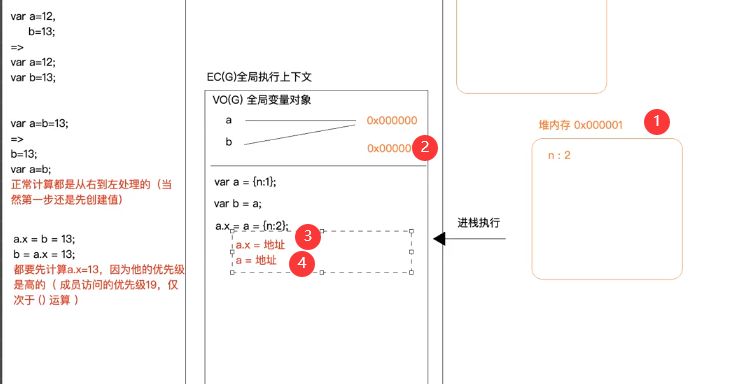1. 开辟内存，假设地址为0x000001
2. 将地址放入栈中
3. `a.x = 地址`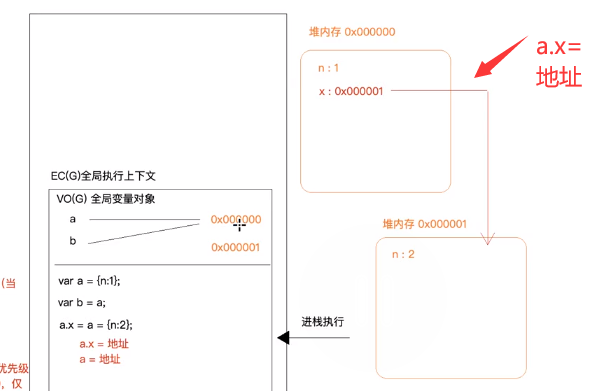4. `a = 地址`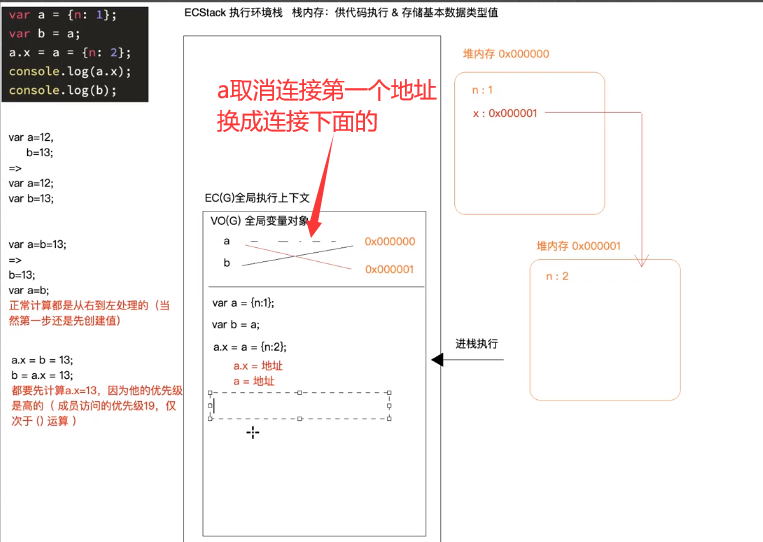`a``{n：2}``b``{n:1,x:{n:2}}`